# Profit And Loss for RRB PO & Clerk | Quantitative Aptitude | Quiz-6

## Profit And Loss for RRB PO & Clerk | Quantitative Aptitude | Quiz-6

Profit and Loss plays a significant role in Quantitative Aptitude Section of banking exams such as IBPS, SBI and RBI PO and Clerk. You will get at least 2-3 questions from Profit and Loss in one of IBPS, SBI and RBI PO & clerk exam. So, aspirants should focus on Profit and Loss questions in detail. Here, we are providing you with the Profit and Loss questions quiz with the detailed solution so that you can easily prepare for Profit and Loss questions. We are providing here all-important latest pattern-based questions and Previous Year Questions of Profit and Loss of various Government Exam like IBPS, SBI, and RBI PO and Clerk exam. This Profit and Loss quiz we are providing is free. Attempt this Profit and Loss quiz to practice important questions with answers and solutions. And score better in IBPS, SBI and RBI PO and Clerk exam.

Profit and Loss Quiz to improve your Quantitative Aptitude for SBI Po & SBI clerk exam, IBPS PO & IBPS Clerk exam , IBPS RRB PO and assistant exam, LIC AAO ,LIC Assistant  and other competitive exam.

1. In place of 18% profit an article is sold at 42% profit and seller gets Rs. 110.40 more. Find the selling price of article if it were sold at 25% profit?
(a) Rs. 440
(b) Rs.460
(c) Rs.575
(d) Rs.550
(e) Rs.5252. The profit earned on selling two articles is Rs. 80 less than profit earned on selling three articles. If 20% profit is earned on selling one article, then find the cost price of the article?
(a) Rs.200
(b) Rs.600
(c) Rs.1200
(d) Rs.800
(e) Rs.4003. A shopkeeper has a stock of rice. 20% of rice is spoiled, then shopkeeper sell remaining rice at 110% of the cost price and weight 200 gram less than 1 kg. Find his overall profit %.
(a) 12%
(b) 14%
(c) 8%
(d) 10%
(e) 4.4%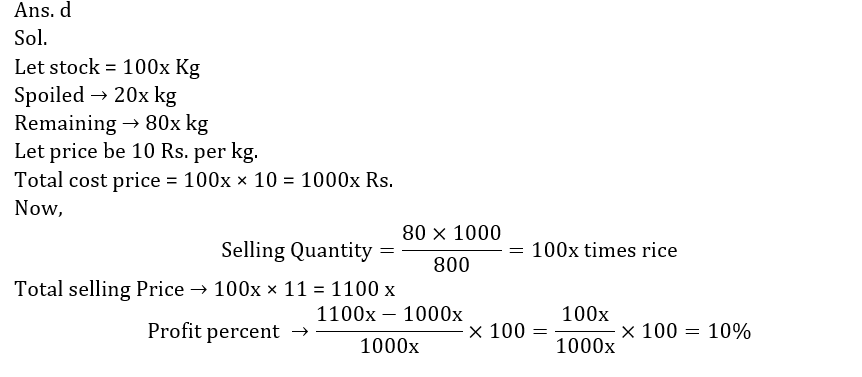4. For every 20-kg retailer give 2 kg rice free. He marked up the rice 50% more than the cost price. A customer came and buy 500 kg rice at 50/3% discount. Find profit percentage of retailer if retailor weighs 20% less?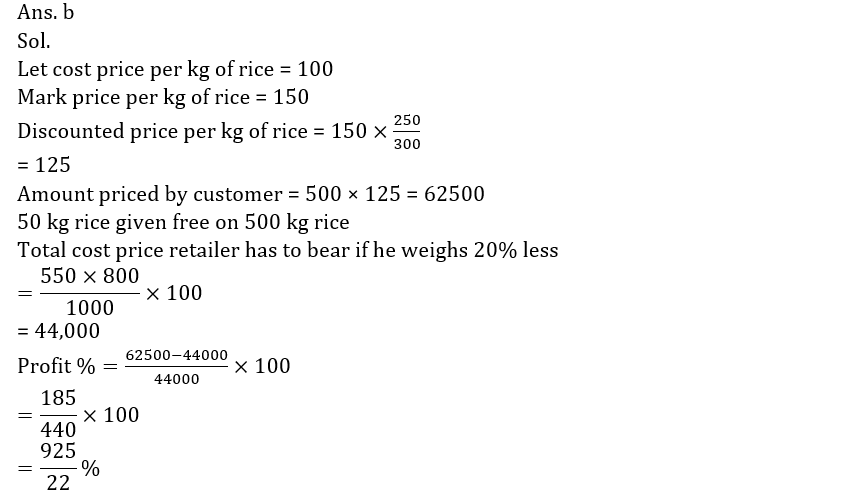5. Ratio between cost price and selling price of a article is 8 : 9 and ratio between numerical value of profit percent and numerical value of profit is 25 : 4. Find the selling price of the article.
(a) 18
(b) 27
(c) cannot be determined
(d) 16
(e) 24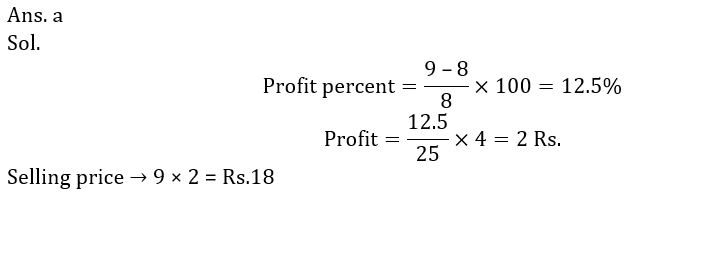6. Marked price of two articles A and B are in the ratio of 7 : 9, at the time of selling shopkeeper gives discount of d% on article A and (d + 5)% on article B and made a profit of 25% on each article, if cost price of article A and B are in ratio 112 : 135. Then find percent of discount given by shopkeeper on both articles?
(a) 12.5%, 17.5%
(b) 25%, 30%
(c) 10%, 15%
(d) 15 %, 20%
(e) 20%, 25%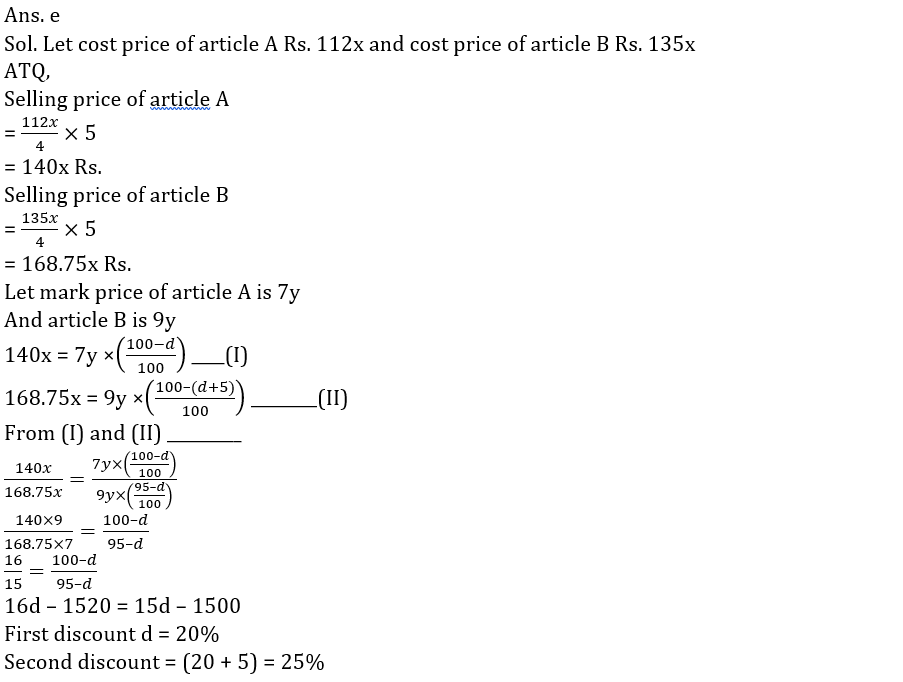7. Ratio between marked price of article A to article B is 4 : 5. Shopkeeper allowed d% discount on article ‘A’ and (d + 18)% discount on article ‘B’, so selling price of both articles become equal. If shopkeeper made a profit of 20% on article A and 25% on article B and profit made on article B is Rs. 384 more than that of article A, then find the cost price of article ‘A’ and article ‘B’ respectively?
(a) Rs.9000 Rs. 8400
(b) Rs.9600 Rs. 9216
(c) Rs.9800 Rs. 9012
(d) Rs.9600 Rs. 8488
(e) Rs.9200 Rs. 9216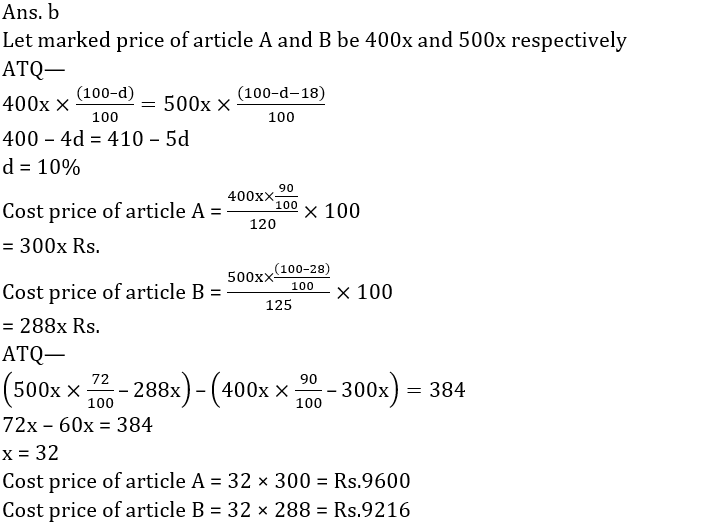8. A garment company declared 17% discount for wholesale buyers. Mr Sameer, a wholesaler bought garments from the company for Rs. 1660 after getting discount. He fixed up the selling price of garments in such a way that he earned a profit of 7% on original company price. What is the selling price?
(a) Rs. 2130
(b) Rs. 2140
(c) Rs. 2410
(d) Rs. 2310
(e) Rs. 2240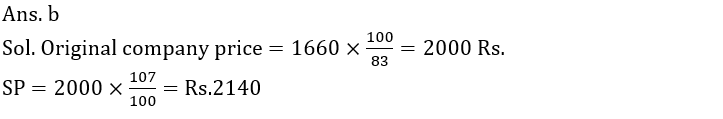9. A person sells his table at a profit of 25/2% and the chair at a loss of 25/3% but on the whole he gains Rs. 25. On the other hand if he sells the table at a loss of 25/3% and the chair at a profit of 25/2% then he neither gains nor loses. Find the cost price of the table and the chair.
(a) Rs. 360, Rs. 240
(b) Rs. 380, Rs. 260
(c) Rs. 400, Rs. 420
(d) Rs. 360, Rs. 410
(e) Rs. 420, Rs. 40010. Selling price of 2.4 kg of rice is Rs. 144 and selling price of 4.8 kg of pulse is Rs. 216 and seller get 20% profit on rice and 25% loss on pulse. Then cost price of one kg of rice is what percent of cost price of one kg of pulse?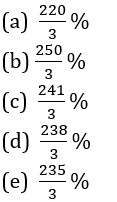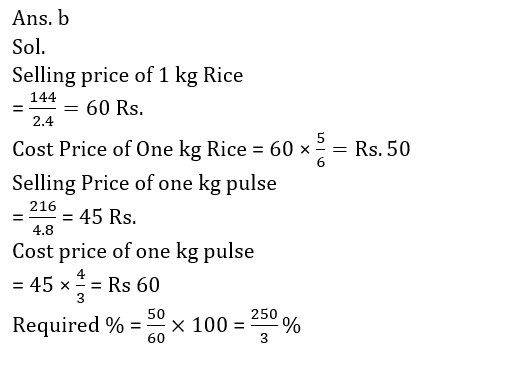Profit and Loss Quiz 6 PDF

#### Attempt Quantitative Aptitude Topic Wise Online Test Series

Recommended PDF’s for :

#### Most important PDF’s for Bank, SSC, Railway and Other Government Exam : Download PDF Now

AATMA-NIRBHAR Series- Static GK/Awareness Practice Ebook PDF Get PDF here
The Banking Awareness 500 MCQs E-book| Bilingual (Hindi + English) Get PDF here
AATMA-NIRBHAR Series- Banking Awareness Practice Ebook PDF Get PDF here
Computer Awareness Capsule 2.O Get PDF here
AATMA-NIRBHAR Series Quantitative Aptitude Topic-Wise PDF Get PDF here
AATMA-NIRBHAR Series Reasoning Topic-Wise PDF Get PDF Here
Memory Based Puzzle E-book | 2016-19 Exams Covered Get PDF here
Caselet Data Interpretation 200 Questions Get PDF here
Puzzle & Seating Arrangement E-Book for BANK PO MAINS (Vol-1) Get PDF here
ARITHMETIC DATA INTERPRETATION 2.O E-book Get PDF here

3